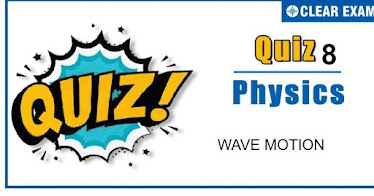## [LATEST]\$type=sticky\$show=home\$rm=0\$va=0\$count=4\$va=0

Q1. There are three of sources of sound of equal intensity with frequencies 400, 401 and 402 vib/sec. The number of beats heard per second is
•  3
•  4
•  1
•  2
Solution
Q2.In Melde’s experiment, the string vibrates in 4 loops when a 50 g weight is placed in the pan of weight 15 g. To made the string vibrate in 6 loops, the weight that has to be removed from the pan in approximately
•  7 g
•  36 g
•  21 g
•  29 g
Solution
As p√T=constant ∴ T_2/T_1 =(p_1^2)/(p_2^2 )=4^2/6^2 T_2=16/36 T_1=16/36×65=29 ∴ Weight to be removed =65-29=36 g

Q3.  Equations of a stationary wave and a travelling wave are y_1=a sinkx cosωt andy_2=a sin⁡(ωt-kx). The phase difference between two points x_1=π/3k and x_2=3π/2k are ϕ_1 and ϕ_2 respectively for the two waves. The ratio ϕ_1/ϕ_2is
•   1
•  5/6
•  3/4
•  6/7
Solution

Q4. The ratio of speed of sound in nitrogen and helium gas at 300 K is
•  √(2/7)
•  √6/5
•  √1/7
•  √3/5
Solution

Q5.Apparatus used to find out the velocity of sound in gas is
•  Kundt’s tube
•  Quincke’s tube
•  Melde’s apparatus
•  None of these
Solution
A

Q6. Three waves of equal frequency having amplitudes 10 mm, 4 mm, and 7 mm arrive at a given point with successive phase difference of π/2 , the amplitude of the resulting wave in mm is given by
•  4
•  5
• 7
•  6
Solution
As phase difference between waves of amplitudes 10 mm and 7 mm is π, therefore, their resultant amplitude =10-7=3 mm. Now amplitudes 3 mm and 4 mm have a phase difference =π/2. ∴ Resultant amplitude =√(3^2+4^2 )=5 mm

Q7.Two trains, each moving with a velocity of 30 ms^(-1), cross each other. One of the trains gives a whistle whose frequency is 600Hz. If the speed of sound is 330 ms^(-1) the apparent frequency for passengers sitting in the other train before crossing would be:
•  600 Hz
•  630 Hz
•  720 Hz
•  920 Hz
Solution
Given, the speed of sound v=330 ms^(-1) Velocity of both trains =30 ms^(-1) (∵v_o=v_s=20ms^(-1) ) And frequency =600 Hz When both trains are moving towards each other then, apparent frequency n^'=n[(v+v_o)/(v-v_s )] =600[(330+30)/(330-30)] =600[360/300] n'=720 Hz

Q8.A device used for investigating the vibration of a fixed string of wire is
•  None of these
•  Sonometer
•  Barometer
•  Hydrometer
Solution
Sonometer is used to produce resonance of sound source with stretched vibrating string

Q9.The source producing sound and an observer both are moving along the direction of propagation of sound waves. If the respective velocities of sound, source and an observer are v,v_s and v_o, then the apparent frequency heard by the observer will be (n= frequency of sound)
•  (n(v+v_o))/(v-v_o )
•  (n(v+v_o))/(v+v_s )
•  (n(v-v_o))/(v+v_s )
•  (n(v-v_o))/(v-v_s )
Solution
D

Q10. Two sound waves (expressed in CGS units) given by y_1=0.3 sin⁡〖2π/λ(vt-x)〗 and y_2=0.4 sin⁡〖2π/λ(vt-x+θ)〗 interfere. The resultant amplitude at a place where phase difference is π/2 will be
•  0.1 cm
•  0.5 cm
•  0.7 cm
• 1/10 √7 cm
Solution
Resultant amplitude =√(a_1^2+a_2^2+2a_1 a_2 cos⁡ϕ ) =√(〖0.3〗^2+〖0.4〗^2+2×0.3×0.4×cos⁡〖π/2〗 )=0.5 cm#### Written by: AUTHORNAME

AUTHORDESCRIPTION## Want to know more

Please fill in the details below:

## Latest NEET Articles\$type=three\$c=3\$author=hide\$comment=hide\$rm=hide\$date=hide\$snippet=hide

Name

ltr
item
BEST NEET COACHING CENTER | BEST IIT JEE COACHING INSTITUTE | BEST NEET & IIT JEE COACHING: WAVE MOTION QUIZ-8
WAVE MOTION QUIZ-8
https://1.bp.blogspot.com/-xR1CyLQtl0k/X6_rIpIsw0I/AAAAAAAANsI/9-zfr6OfGRsxLXBVNzWSLU9xwdbYz2A2gCLcBGAsYHQ/w374-h221/Copy%2Bof%2BQuiz%2BImage%2BTemplate%2B%25281%2529%2B%252828%2529.jpg
https://1.bp.blogspot.com/-xR1CyLQtl0k/X6_rIpIsw0I/AAAAAAAANsI/9-zfr6OfGRsxLXBVNzWSLU9xwdbYz2A2gCLcBGAsYHQ/s72-w374-c-h221/Copy%2Bof%2BQuiz%2BImage%2BTemplate%2B%25281%2529%2B%252828%2529.jpg
BEST NEET COACHING CENTER | BEST IIT JEE COACHING INSTITUTE | BEST NEET & IIT JEE COACHING
https://www.cleariitmedical.com/2020/11/wave-motion-quiz-8.html
https://www.cleariitmedical.com/
https://www.cleariitmedical.com/
https://www.cleariitmedical.com/2020/11/wave-motion-quiz-8.html
true
7783647550433378923
UTF-8

STAY CONNECTED